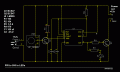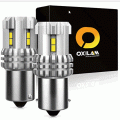# Transistor datasheets 210503

#### allenpitts

Joined Feb 26, 2011
155
Hello AAC forum,
Working on circuit that is triggered by a mini PIR (HC-SR505)
and, using a 555 timer, turns on some LEDs for a certain time.Was working with 9 volts using 5mm thru-hole LEDs but even
30 5MM LEDS would not light a walk-in closet. So research
LED to Oxilam 1136-12k-W2-ous. A 5mm thru-hole LED puts out
about 4-5 lumens. The Oxilam, which is an automotive back-up
light, generates 2800 lumens. Four of them will more than light
a closet.So to accomodate the 12 volt automotive Oxilams the power supply
was changed from nine volt to twelve volt. And after the research
was done it was realized that Q1 is operated, by the PIR, and
Q2 is operated by the output of the 555. So neither are connected
directly to the 12 volt supply.

But in doing the research these questions were asked.

1a. What is the maximum voltage that the BC547 would tolerate
at the base? At what point does the transistor get fried?

1b. What is the maximum current the BC547 will tolerate
at the base. (Perhaps the BC547 base will not draw
more current than it can tolerate?)

1c. What is the maximum current and voltage that can be passed from
the BC547 common to the emitter?

2a. What is the maximum voltage that the would tolerate
at the base? At what point does the transistor get fried?
BD437g
2b. What is the maximum current the BD437g will tolerate
at the base. (Perhaps the BD437g base will not draw
more current than it can tolerate?)

2c. What is the maximum current and voltage that can be passed from
the BD437g common to the emitter?

The datasheet for transistors BC547 and BD437g are attached herewith.

One tract suggested that the dissipation rating of the device
be observed. This parameter tells how much power the transistor can safely handle
without being damaged. Transistors are typically described as power or small-signal,
dependent on the value of this rating. Power transistors typically can dissipate a watt or more of power,
while small-signal ones dissipate less than a watt. The maximum dissipation for a 2N3904 (BC547 equivalent)
for instance, is 350 mW (milliwatts), and so it is classified as small signal.

But nowhere on either of the transistor data sheets can something
called 'dissipation rating' be found. (Power dissipation?)

Also found some literature that says:
'The breakdown voltage is where the transistor will stop operating or be destroyed if it is given an
input voltage of that amount. (It is thought that this is addressing question 1c above.)
Vcb is the voltage between the collector and the base. Vceo is the voltage between the collector and
the emitter with the base open, and Veb is the voltage from the emitter to the base.
The Vcb breakdown voltage for the BC547 is listed as 50 V. The remain
ing values are 45 V for Vceo, and 6 V for Veb. These are amounts that should be avoided in actual operation.

For the BD537g the Vceo looks like 45 v which would be the answer to question 2c above and would handle
the twelve volts at Q2.

And I think, in the BC437g data sheet the parameter marked 'Ib Base Current' the value is 1A (amp) which
is the answer to 1a. above.

You pardon is begged for the length of this query. It is hoped that its length may be ameliorated by the
explicitness of the questions.

Thanks

Allen Pitts, Dallas TX

#### sghioto

Joined Dec 31, 2017
3,315
The LED bulbs draw 440ma each so 4 bulbs is 1.76 amps. You will need a 12 volt supply rated at least 2 amps.
A BC547 is only rated at 100ma so you obviously need a higher current device.
I recommend a N channel mosfet such as the SPT35NF10 rated 40 amps at 100 volts.
Replace Q2 with the above device.

#### MisterBill2

Joined Jan 23, 2018
12,391
Transistor ratings usually have both maximum and typical ratings, and it is important to avoid exceeding any of those max ratings. So for a power switch, both voltage and current rating must not be exceeded, as well as the maximum power dissipation, which can be calculated as the product of the load current and the saturation voltage at that load current.
For a reasonably sensible design allowing a safety margin of 20% to50% is reasonable for lower power systems like this.
And it might make more sense to use LEDs not rated for 12 volts, and putting them in series. That could allow the same current as a single device, which might allow a smaller control transistor.

#### crutschow

Joined Mar 14, 2008
30,086
1a. What is the maximum voltage that the BC547 would tolerate
at the base? At what point does the transistor get fried?
1b. What is the maximum current the BC547 will tolerate
at the base. (Perhaps the BC547 base will not draw
more current than it can tolerate?)
There is no particular maximum Vbe rating, the base-emitter junction looks like a forward biased diode with voltage of about 0.6 to 0.7V at typical operating currents.
The base-emitter will draw all the current you provide it until you zap the junction.
You need a resistor in series with the base to limit the current to comfortably below its max rating.

The transistor is only rated for a 100mA collector current so it's maximum base current is likely well below that.